# 1- Water is pumped through a 9 m long pipe with internal diameter of d-40 mm at the rate of 2.25 m3 per hour. The water inter the pipe at 30 °C and exit at 70°C. Determine: b) The convection heat tra...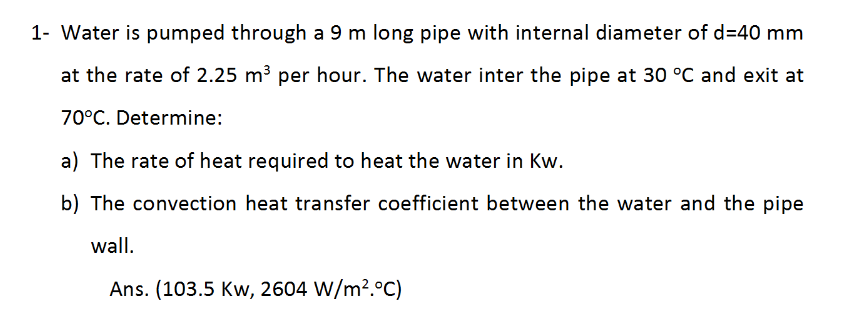1- Water is pumped through a 9 m long pipe with internal diameter of d-40 mm at the rate of 2.25 m3 per hour. The water inter the pipe at 30 °C and exit at 70°C. Determine: b) The convection heat transfer coefficient between the water and the pipe wall Ans. (103.5 Kw, 2604 W/m2.°C)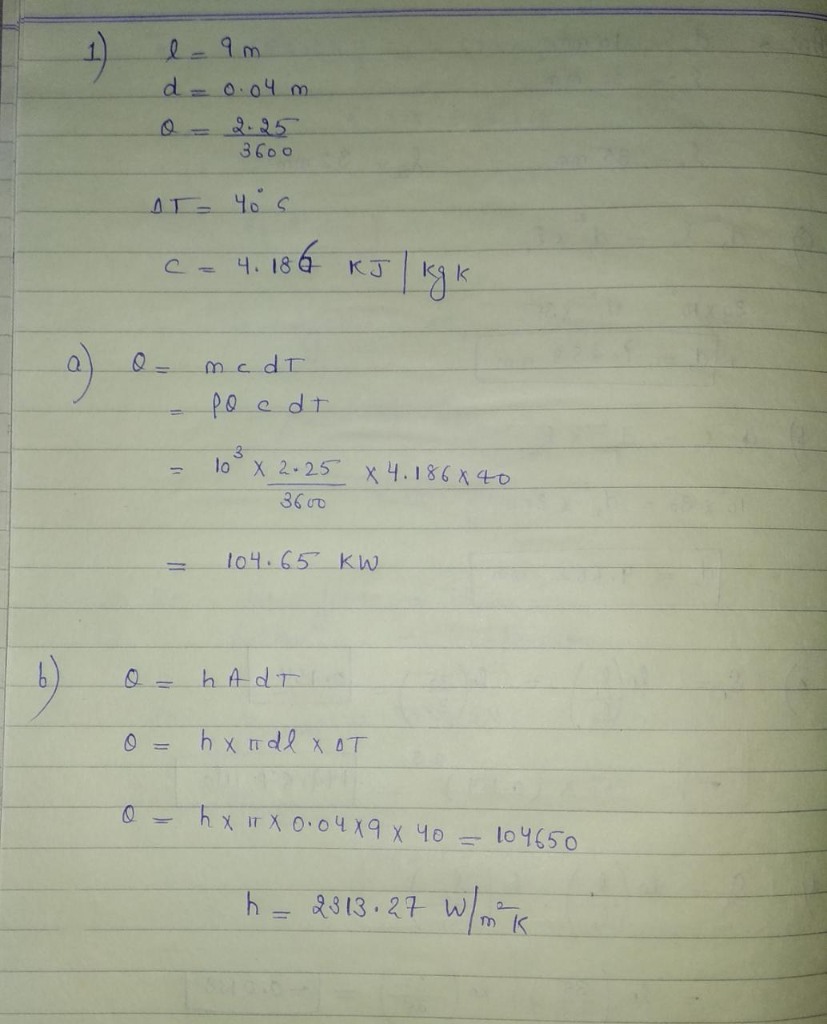##### Add Answer of: 1- Water is pumped through a 9 m long pipe with internal diameter of d-40 mm at the rate of 2.25 m3 per hour. The water inter the pipe at 30 °C and exit at 70°C. Determine: b) The convection heat tra...
Similar Homework Help Questions
• ### Help mee!! Q1. Heat conduction & convection (38 pt). Hot water flows in a steel pipe...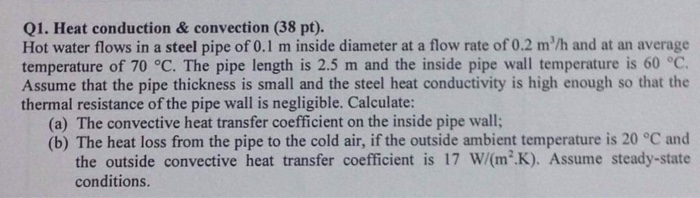Help mee!! Q1. Heat conduction & convection (38 pt). Hot water flows in a steel pipe of 0.1 m inside diameter at a flow rate of 0.2 m/h and at an average temperature of 70 °C. The pipe length is 2.5 m and the inside pipe wall temperature is 60 °C Assume that the pipe thickness is small and the steel heat conductivity is high enough so that the thermal resistance of the pipe wall is negligible. Calculate: (a) The...

• ### 4 Air (with density 6.08 k3 and viscosity coefficient 2.95 x 10 Pa.s) at an average pressure of 10 bar and a bulk temperature of 600 K flows through a 0.5 m long, 20 mm internal diameter circular tub...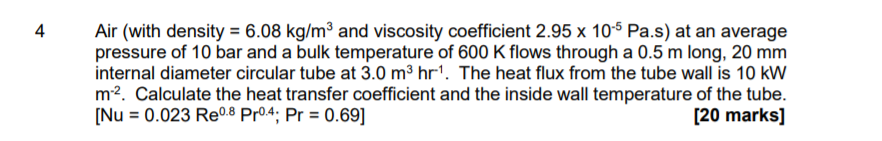4 Air (with density 6.08 k3 and viscosity coefficient 2.95 x 10 Pa.s) at an average pressure of 10 bar and a bulk temperature of 600 K flows through a 0.5 m long, 20 mm internal diameter circular tube at 3.0 m3 hr1. The heat flux from the tube wall is 10 kW m2. Calculate the heat transfer coefficient and the inside wall temperature of the tube. [Nu0.023 Re08 Pr04; Pr 0.69] [20 marks] 4 Air (with density 6.08 k3...

• ### heat and mass transfer (20 points) Air at 25°C cools a 2170 cylindrical battery (with a diameter of 21 mm and a length of 70 mm) with a cross flow velocity of 6 m/s. a) Determine the average conv...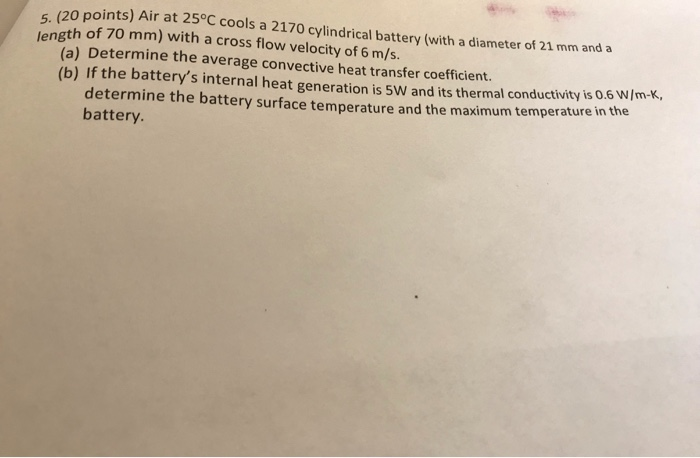heat and mass transfer (20 points) Air at 25°C cools a 2170 cylindrical battery (with a diameter of 21 mm and a length of 70 mm) with a cross flow velocity of 6 m/s. a) Determine the average convective heat transfer coefficient. (b) If the battery's internal heat generation is 5W and its thermal conductivity is 0.6 w/m-k, the determine the battery surface temperature and the maximum temperature in battery (20 points) Air at 25°C cools a 2170 cylindrical battery...

• ### 8-C) As shown below water is pumped and sprayed through a nozzle into the atmosphere at 20 m/s at...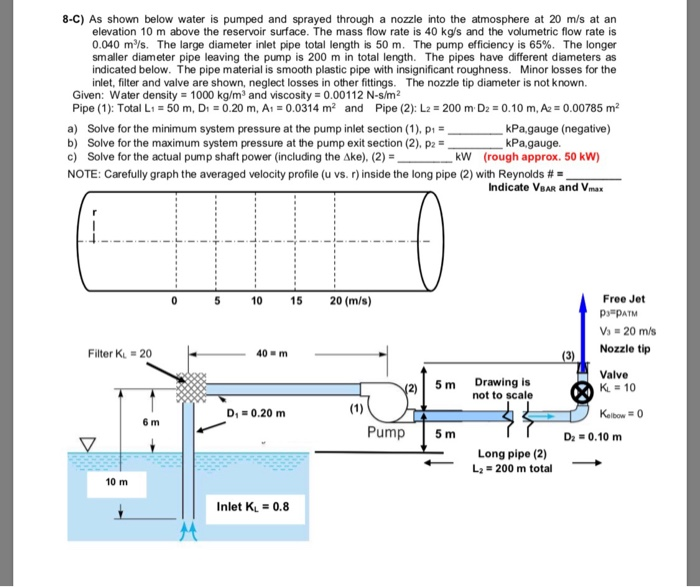8-C) As shown below water is pumped and sprayed through a nozzle into the atmosphere at 20 m/s at an elevation 10 m above the reservoir surface. The mass flow rate is 40 kg/s and the volumetric flow rate is 0.040 m3/s. The large diameter inlet pipe total length is 50 m. The pump effidency is 65%. The longer smaller diameter pipe leaving the pump is 200 m in total length. The pipes have different diameters as indicated below. The...

• ### 1. At an outdoor lot, there is a black-colored solar heat collection copper pipe. It is 50 meters...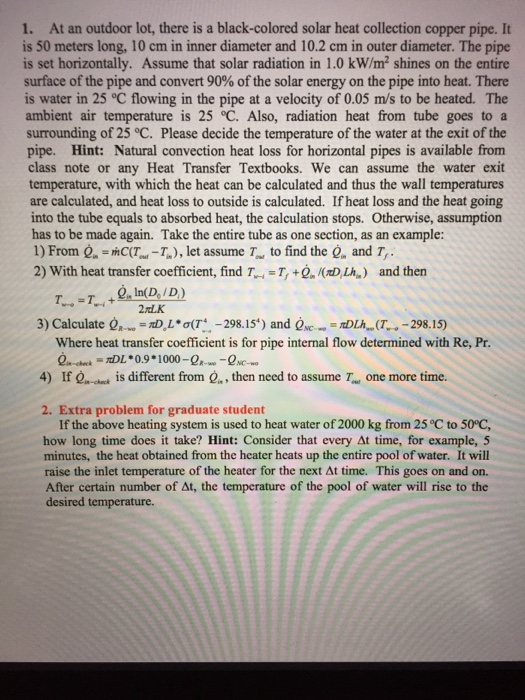Please answer question 2. Quastion 1 answer is already provided in the photos. 1. At an outdoor lot, there is a black-colored solar heat collection copper pipe. It is 50 meters long, 10 cm in inner diameter and 10.2 cm in outer diameter. The pipe is set horizontally. Assume that solar radiation in 1.0 kW/m2 shines on the entire surface of the pipe and convert 90% of the solar energy on the pipe into heat. There is water in 25...

• ### Problem 2: A stainless steel rod (k-21 W/m-K, p-8000 kg/m3, C,,-570 J/kg-K) with diameter D-10 mm is heat-treated as it...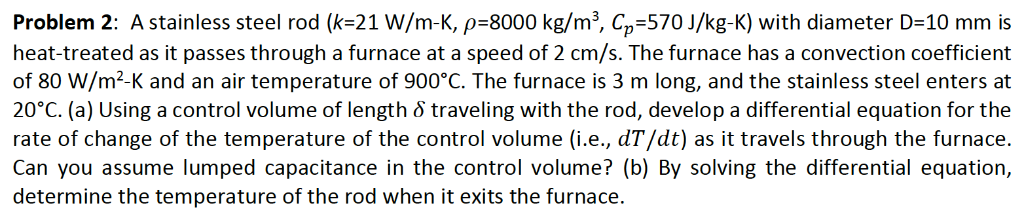Problem 2: A stainless steel rod (k-21 W/m-K, p-8000 kg/m3, C,,-570 J/kg-K) with diameter D-10 mm is heat-treated as it passes through a furnace at a speed of 2 cm/s. The furnace has a convection coefficient of 80 W/m2-K and an air temperature of 900°C. The furnace is 3 m long, and the stainless steel enters at 20°C. (a) Using a control volume of length S traveling with the rod, develop a differential equation for the rate of change of...

• ### Water at 15°C (ρ  = 999.1 kg/m3 and μ = 1.138 × 10−3 kg/m·s) is flowing steadily in a 34-m-long and 6-cm-diameter horizontal pipe made of stainless steel at a rate of 10 L/s. Determine the pressure d...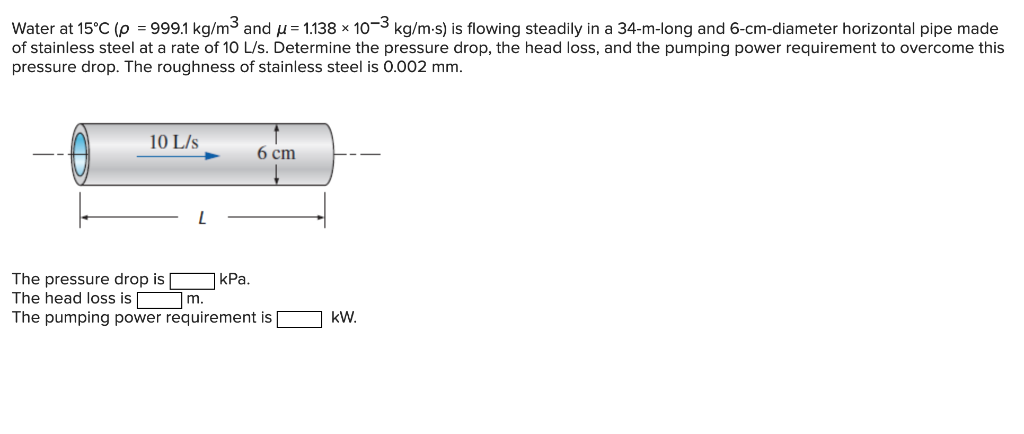Water at 15°C (ρ  = 999.1 kg/m3 and μ = 1.138 × 10−3 kg/m·s) is flowing steadily in a 34-m-long and 6-cm-diameter horizontal pipe made of stainless steel at a rate of 10 L/s. Determine the pressure drop, the head loss, and the pumping power requirement to overcome this pressure drop. The roughness of stainless steel is 0.002 mm. Determine the following: A)The pressure drop in kPa. B)The head loss in m. C)The pumping power requirement in  kW. Water at...

• ### Homework 7 heat transfer 2018-20 1) A horizontal tube of 12.5-mm dimeter with an outer srface temperature of 240°C is placed in a room with an air temperature of 20°C. Estimate the heat transfer rate...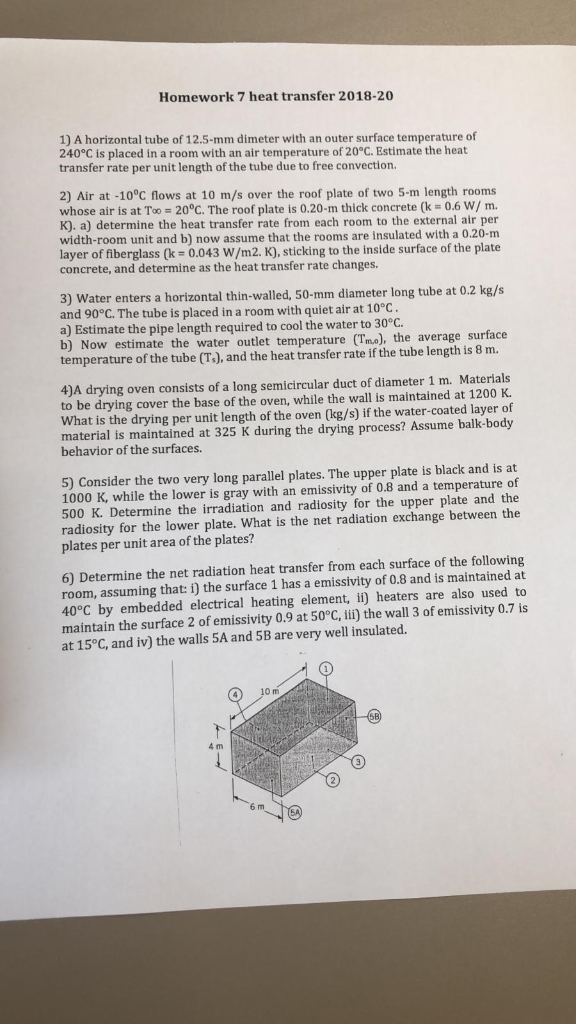Homework 7 heat transfer 2018-20 1) A horizontal tube of 12.5-mm dimeter with an outer srface temperature of 240°C is placed in a room with an air temperature of 20°C. Estimate the heat transfer rate per unit length of the tube due to free convection. 2) Air at -10°C flows at 10 m/s over the roof plate of two 5m length rooms whose air is at Too-200C. The roof plate is 0.20-m thick concrete (k = 0.6 w/ m. K)....

Need Online Homework Help?